#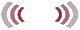WAVE SPECTRUM

CHAPTER 30              June 1998.INDEX TO OTHER PAGES

1. This is a pro and con session into the nature of what is called the “magnetic” spectrum.  Our aim will be to list that which we know to be facts, and question them for their how and why along with everything else.

2. So that at end - when we have learned more in the nature of these waves than ever before - we may come to the conclusion - to know less about them than we do now.

3. Right now we seem to be quite at ease with what we know of light and the rest of the spectrum, as if we have it all neatly tucked-in.  Everything is mathematically accounted for, nor do we question our values because having worked so hard we do not want to see error.

4. Electricity is not a wave at all, just electrons going for a hay-ride, and the word “justification” having been omitted from our dictionaries we can safely list this none-wave phenomena as part of the wave spectrum.  And if anyone remarks about this contradiction, we simply do not accept criticism.

5. As then most of us use a civil tongue, there are those whose language is atrocious, like “how would you like to include pairs of perceptually confusable objects, or transferase, to name some.

6. And what I deem as a bad habit is; to name everything after the man that thought it out, so if you want to compute Charles you divide Henry, with Derickson, to the outcome of Guass, in the Frederick equation.

7. Photons, as man has devised, are of course none existent, these things are no more than imagination along with the so called charges, or lose electrons, or vibrating electrons, or jumping electrons all of which is mere fabrication in the imagination of men lacking knowledge in fundamentals.

Fundamentals

1. Emphasizing a rule to ourselves - is for all things in motion to adhere to the laws thereof, for particles as for waves, and that if it does not comply with these laws we shall be in error.

2. And my rule, never to trust man's interpretation of his experiments.  For as we have seen with the interferometer in its null result (Figure 30-1 leading to that folly of relativity.   And with the diametrically opposite star experiment (Figure 30-2), in its so-called failure.

3. Both serve us with evidence that light for its movement and for its velocity is un-mistakenly “dependent” on our physical media and upon the movement of that media.  Which is by a circular mode of propagation as evidence has shown.

PROPAGATION OF LIGHT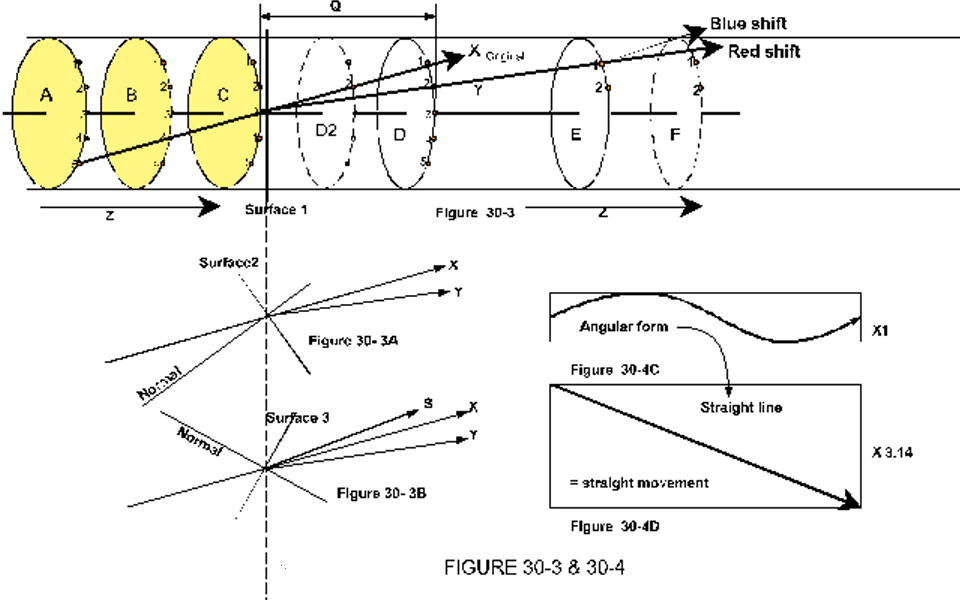1. Having previously shown the evidence noted above, let us reaffirm them taking a different approach.  Illustration figure 30-3, demonstrates a section of a light-wave as it passes a number of atoms from A to F on the basis of a circular mode.

2. In the first section (atoms abc), the wave passes from 5 O’clock to 3 O’clock after which it encounters a change in density similar to that area just above a roadbed on a hot summer day when the road appears to have a wet surface.

3. The change therefore is from air to air at normal temperature to a greater temperature wherein the atoms are spaced further apart (lower density).  By surface 1, a normal (straight on) direction will cause the angular incidence to connect upon 2 O’clock of atom D, which is now at the D2 position.

4. As in fact it does connect upon the 2 O’clock mark of atom D at its D2 position causing the angular component of the wave to change proportionally to the expansion, the greater spacing of the atoms.

5. The how and why comes to a conclusion that; yes, the wave is dependent upon the media to cause it to change for no better reason than a spacing of atoms.  For we know it to be a fact that from 3 O’clock on atom C, the wave will change its angular direction to Y, accounting for a red shift.

6. So we ask; what is there in area Q to cause the wave to change?  It seems that while the wave was supposed to go from 3 O’clock C to 2 O’clock D, - it is indeed absolutely determined to do so regardless of the greater spacing.

7. The suggestion here is; that it is not in the power nor in the directive of the wave to follow a path, but in the atoms along which it passes, proving how very dependent the wave for its propagation in the angular component is upon the media.

8. This does not necessarily imply that the direction of propagation (direction Z) has changed.  On the contrary, coming from the normal, the wave passes straight on through, with the only change being upon the angular component of the wave.

9. While we spoke as if it was not in the power of the wave to decide for its direction of travel.  (The atoms by their relative spacing reshaping that direction).  It leaves us with the quest as to how it comes to travel in such perfectly straight lines over enormous distances that are not necessarily around a row of atoms placed neatly in front of its trajectory, but over atoms positioned at random.

10. And not by a diameter the size of any single atom, but by a diameter within which a number or numerous atoms may be contained.  And how to explain that?

11. In my judgment, considering how everything in nature is twofold, or by twofold, the direction of propagation (arrows Z) (as one of the two factors), is unique in respect to its relative factor, the waveform, which in itself is also unique.

12. Therefore, that factor (as factor # one) is the very essence of speed in the twofold factor of velocity and in the revolutions to give it inertia.

13. This in turn, by the laws of motion, cannot but must travel a straight line unless diverted by an equal action wherein the spacing of the atoms does not represent the essence of speed nor the consequential inertia.

14. But it does represent that factor called angular propulsion by which it is bound to the media, within which the angular component of the wave lies embedded.

15. These factors are evident by the two previously named experiments how the velocity remains constant upon any media in whatever movement that media may be found, and in our experience to the straight-line trajectory of light.

16. To ask, how does the wave come to this velocity?  The answer in the twofold factor of velocity, in the relative part thereof (distance in time), is easily known from measurements.

17. Or, mathematically established from the first factor known as its velocity of constant - as the speed at which the wave by its angular component moves around the circumference of the circular formation.

18. A wave moving around a circumference, or by any sine formation, passes more distance compared to the same moving in a straight line.

19. Any part of the wave therefore  (whether or not we assume it by the immaterial essence of a sine formation, or by a more material sine formation such as (for example only) a stream of finite dust particles on the move) that part moves at all times at a velocity never less and never more than 300.000km/sec.  Wherefore it is termed the constant of, noted Vc. Velocity constant.

20. The second factor of the twofold nature in the propagation of waves, the angular momentum, which for the moment we shall assume to be drawn and propelled by the angular moment of the atoms (electron movement), is for all of its angular moment still found to travel by a straight line (trajectory).

21. This is demonstrated by figure 30-4 / D wherein the tube is laid out in its circumference, showing the movement to pass straight on while it follows its angular momentum around the tube. (Figure 30-4-C)

22. The angular track is thus without deviation to the right or left, which is not true, nor could it be true for a transverse wave - as one factor in agreement with the laws of motion for the circular mode.

23. Summarizing the wave in its twofold being; there is a "power" and a "directive" that is of the wave itself, namely; the essence of its speed into inertia, and the factor where its form and movement are of the media.

24. And so in our objective to fundamentals we cannot look upon a wave in singular terms.  For while we are of a mind to say; Yes I can see how and why the wave is so dependent on the media, experiments attesting thereto.

25. There is also this fact that it seems independent of, or not susceptible to the media in the fact that it goes by a straight trajectory, as if the precise locations of atoms, and/or molecules is irrelevant to it.  The answer thus must for the moment reside in its twofold factor.

26. If we take surface 1 (Figure 30-3)  and change the angle to surface 2 so that the wave comes to strike at an angle out of the normal.  The wave must refract to provide us with the illusion of a wet roadbed.

27. The rule then for the wave is to refract away from the normal.  (Meaning also it comes from the interior or else it would turn towards the normal).

28. Thus incidence X, does what the illustration shows refracting into direction y.  But while (y) in the illustration is the angle by the shift, in 3A it is the angle in refraction, and both are equal since the shift as well as refraction are at all times proportional to the index of the composition.

29. If then we change the angle of the surface to surface 3, (Figure 30-3B), the angle of refraction will be S, while the shift remains y.  It is thus mere coincidence that angles x/y appear the same in figures 30-3 and 30-3A. since figure 30-3 can be shown in any other position of the wave around the circumference of the atoms.

VELOCITY OF LIGHT

1. Having assumed the velocity of constant to come of and by the media, it would be simple to state that Vc is by the electrons in their angular momentum.  For what else is there in the atoms or between the atoms in our illustration figure 30-3 that propels the wave from one to the next?

2. Indeed, what else?  The else is that there is more to consider, a greater knowledge at which I am not at liberty to go into.

3. And so just as we assume the material part of the atom to be material even though we do not have any conception as to what "material" such may be, or immaterial for whatever that may be. Likewise it is enough for man to visualize the propulsion factor of light of and by the angular atomic momentum.

4. Ironic that I should be reproving man for his immaturity to believe upon the stork, and yet here I am teaching and confining them to the same. The only difference being; man believes upon the Stork for ignorance, I refer them to it for better knowledge, or because they are yet babes not mature enough for the full reality.

5. A red wavelength like unto a high speed drill-bit on the move accounts for its stability and straight line trajectory, but this does not explain its speed, much less the constant thereof when it is said (or presumed) that electrons have a velocity of only 30,000 km/sec.

6. And if we liken the propulsion to our accelerators to boost the part each time it comes around for its ultimate velocity, how is this to be applied to light?

7.   For while in our accelerators we are boosting particles with an existing velocity of 300,000 km/sec, how is a message at the atoms to be transposed faster than the velocity at which it is handed from one to the next?  So much thus for our electron theory in the nature of electricity.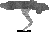WAVE VELOCITIES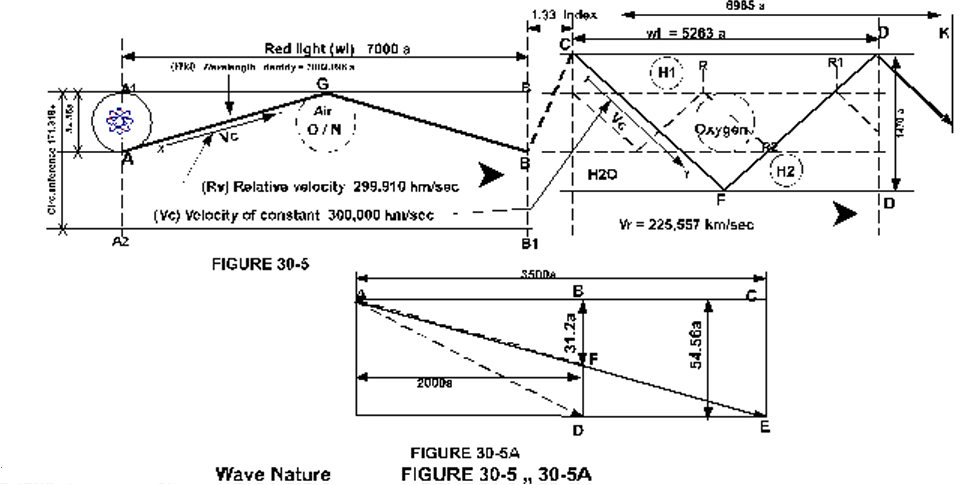1. This heading is plural, for as the velocity of constant (Vc) figure 30-5, x to y is the same for all waves, it is only that which moves without wave-form that could come to that velocity by a measure of distance in time.

2. Accordingly, each and every wave must have a reduced velocity proportional to its length by the diameter or amplitude of its waveform.

3. Distance A1B, figure 30-5, is 7000a (a = unit of angstrom, 0.1nm), which for the light traveling AGB by a diameter of 54.56a comes to a full-length identity (w’id) of 7002.096a.  This by Vc for the wave comes to a relative velocity (Vr) of 299,910 km/sec.

4. Thus, it is no longer sufficient to utilize c as the notation for the velocity of light in the fact that wave velocity is a twofold factor,  For this reason c becomes Vc.  And Vr the notation for the relative velocity by which the speed of light is found distance in time.

5. We must also set a standard for the identity of the wave, considering that a wave from the moment of its inception will retain its identity, which may be assumed as its full length AGB, rather than wavelength AB.

6. For if AGB should change at any time, a red wave might become green, nor therefore would we have our spectral identity or analysis into a shift to either direction.  As therefore the notation for wavelength be w’l, the identity should be w’id, and for the diameter w’di.

7. The next query is; how did we come to that relative velocity and to that measure of the identity of the wave, and its diameter, to find the w’di?  I was forced to learn trigonometry, finding an unknown by two knows, or even two unknowns by one known.

8. Accordingly, I divided the velocity by wavelength to obtain frequencies, and dividing the same into Vc gave us the w’id at 7002.096a.  And the diameter of the wave by rolling the tube into a flat triangle, of which the two known lengths were 7000a and 7002.096a, the unknown, w’di, came to 54.56a.

9. For with anything traveling by a circular path, we should add the circumference to the length, since the wave passes by that factual distance.  And placing the circumference on a two dimensional plane, by figure 30-5

A1A2 = square root of A1B12 minus A1B2

A1A2 = 7002.0962 - 70002 = >>>sqrt = 171.3184 : 3.14 = 54.56a

Confirming 70002 + 171.31842 = >>>sqrt = 7002.096a

WAVE COMPARISON

1. And for a comparison to a blue wave at 4000a traveling at the same relative velocity, comes to w’id at 4001.2a, having a circumference of 97.9869, and diameter at 31.2a.  And so we must admit that it serves us well to make comparisons if for no other reason then to verify our results, since in this case we are in error.

2. The reason is simple, if the above figures were correct - we would not have the dispersion of light into its colors, in the fact that then the tangent for the angular component would be the same for both waves, red and blue.

3. Evidence to this may be seen by figure 30-5A, wherein AC is the half-wave measure of the red, and CE its diameter.  If then we overlay the blue wave by its half-wave AB measure at its BF diameter of 31.2a, its angle AF is identical to red at AE.

4. Accordingly, the evidence (in that the blue must have a proportional greater angle by which the colors are separated in refraction) points out that the same cannot be traveling at the same relative velocity to that of the longer red wave.

5. And that the added measure in its diameter must be according to FD in figure 30-5-A  which is that measure in the angle of the wave proportional to the lengths thereof, giving the blue wave a diameter that is identical to the red wave.

6. Thus to find the measure of the true identity of the blue wave by the formula of the triangle (figure 30-5A)  AB, and BD, being known, the AD measure would come to 4003.667a.

7. Accordingly, since in 300.000 km/sec there can only be 74,931.3067 meter lengths of blue waves, the relative velocity will be 299,725 km/sec, 185km/sec slower to the red.

8. It is thus evident that light for its various wavelengths travel at identical tubular width within a single given medium.  And if we compare this to what is reported under a heading of polarization that by an increased number of lines the longer lengths may be filtered out leaving the shorter lengths to pass, we must give it an "F" rating.

9. Since if indeed blue wavelengths passed by proportionally smaller amplitudes a spectra would record all wavelengths on one single point, something which we factually know not to be.

10. Nor therefore would any wavelength refract to any diverging direction, which by looking at the rainbow we also know not to be so.

11. Accordingly, our grading to an F, for that statement under polarization must stand unless there is an alternative complimenting both - that for the moment as yet remains illusive.

WAVE COMPRESSION

1. We may as well go into measures to see what may be found if any to give us a better understanding ultimately leading to the answers of our search.  To thus find the measures of the wave by figure 30-5, as it is blue shifted into the water by an index of 1.33.  7000 divided by 1.33 comes to a new wavelength (CD) of 5263a, having a new relative velocity at 225,557 km/sec. Length CFD remaining the same since it is assumed the identity of the wave.

2. And here again finding the unknown leg of the triangle by the square root of the sum of the square of the two known legs.  This comes out to a measure of 4618.45958 as the circumference divided by 3.14 = 1470.847a as the diameter by which this red wave must pass along the H2O molecules.

3. This would make the new diameter about 27 times as great, and is that conceivable, or shall we be in error? I deem it in error.

4. Suppose the diameter increased by 2.33 and the wavelength held its wave-crest to wave-crest measure by the index of 1.33, it would bring the identity of the wave to span CFDK, and what would that do to our relative velocity?

5. By 52632 plus 54.56 x 2.33 x 3.14 = 399.182 = 5278 subtracted from 7002.096 = 1724 + 5263 = to bring wavelength CK to 6985a, with its Vr at 299,357 km/sec.

6. Accordingly, there is too much wrong here, for how can we speak of an index at 1.33 and wavelength at 5263a when the light has entered the water for a distance of 6985a, which cannot but must be interpreted into a relative velocity of 299,357 km/sec?

7. The alternate to this is, assuming the diameter and identity of the wave to remain unchanged that the wave is simply compressed like unto a coiled spring.  In that case it takes on the shape of the broken line in figure 30-5, to wrap itself more around the tube.

8. The identity of the wave is then indeed contained within the so-called wavelength CD noted at 5263a, but its crest to crest measure is not anywhere near 5263a.  If this were the case then there be two wave-lengths, the one mentioned, and the R to R1, crest to crest measure.

9. We know that CFDK cannot exist, the velocity being too great for the actual velocity obtained by experimental data.  The wave must therefore increase in diameter (in this case) 27 times over to maintain a crest to crest wavelength at 5263a.  Or, simply be compressed within its existing diameter.  Whichever one the case may be.

10. This must be left to experiment since for the moment considering alternates, if a compression upon an expanding diameter were the case, the wave angle would be different.  While in a compression upon a none expanding diameter - the wave angle shows to remain the same (angle C/F identical to angle R/R2).

11. A spectral shift will thus show the same for both, wherefore in that way we cannot tell the difference.

Cause not in velocity

1. Speaking for velocity, the evidence is unassailable that light cannot possibly slow down within any density in order to provide us with a relative (Vr) measure of the light.  We are of course speaking of the velocity of constant, the real velocity at which light is transposed, all other velocities being incidental, a variant product of distance in time.

2. For if the light did not pass from C to F to D, or AGB at its full 300,000 km/sec we would never come to a relative velocity in water at 225,557 km/sec, nor in air at 299,910km/sec.

3. Consequently, shorter waves do not travel slower, but for their length are forced to travel more around the circumference (or, in the angular component) wherein the relative velocity of the blue wave is found less to that of the red.

Susceptibility

1. If magnetic waves were not duly susceptible to the atoms and molecules of our physical media why should they refract to any index of retardation?  Or why should they turn just inches from a hot roadbed to make for a wet appearance?

2. If light did indeed move independent - the interferometer (Figure 30-1) would have given us a positive result, and Mr. Michelson would have recorded different velocities for the speed of the light of his two stars (Figure 30-2).

3. There is no question about that.  But it did not happen, these two experiments together with what we have seen so far clearly show light to move by a mode that is dependent on the physical media, and to the movement and density thereof.

4. The foolishness of light in space traveling at the same velocity for every observer is a childish thought.  For we are observers, and knowing our earth to be moving while Michelson read the light at near Vc, to us mature persons the light in space traveled conclusively at a greater velocity than the speed of light.

5. Einstein therefore and those that concurred with him were speaking for their own in-mature outlook.

6. This may be seen as a strong statement for all that allowed themselves to be diluted with that folly.  But my reply thereto is; if the shoe fits let him wear it, for these things are such for which no great knowledge or insight is required to comprehend them for their reality.

7. For again what foolishness is it to conclude that the light of a star as it grazes the sun is bend by gravitational force?  For we know how any atmosphere for its density acts as a prism, like the sun being below the horizon when in fact we are already able to see it rising.

8. And thus the light of the star being refracted by the sun’s prism - how is that gravitational?  We are working earnestly with all diligence to come to a understanding of the fundamentals, and we all make errors, and we are not therefore to be disregarded, but of what value are such as clearly depart from reality?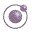Wave  movement how?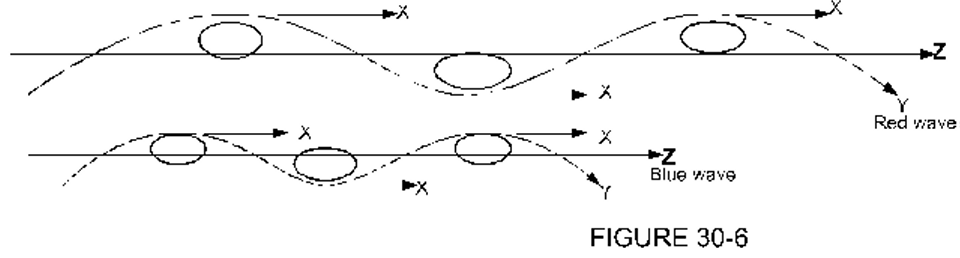1. Our current version in the transmission of waves is like unto a train wherein all the boxcars follow the locomotive depicted by figure 30-6, wherein Y is the locomotive following the sine of the track, with its trajectory from place to place by arrows Z.

2. If then for an alternate we assume no angular movement within the wave, so that every part of the wave moves by X, and Z the linear component only, how will that correspond with experience?

3. The answer is; if our data for blue light to travel slower to the longer lengths is correct- then it will not, since in that event the blue wave will have a relative velocity equal to that of the red.

4. Nor would there be a twofold factor in the velocity, the velocity of constant being the same as the relative velocity.   Or, for another alternate, assuming the wave to move as pulses upon a string, (Figure 30-6-M), a mechanical emulation.

5. The atoms or molecules of the media would have to move perpendicular into the sine formation by distance R.  And what might be the velocity (V) by which this may be transposed?

6. It seems obvious that a velocity limited to the movement of the media is not likely to come to the speed of light.  For considering how sound comes to a velocity of some 1000 m/sec by no more than a fractional movement of the atoms, a movement of atoms into the full width of the amplitude would certainly be less.

7. Thus we shall continue with our current understanding in the propagation of magnetic waves.

Angles of refraction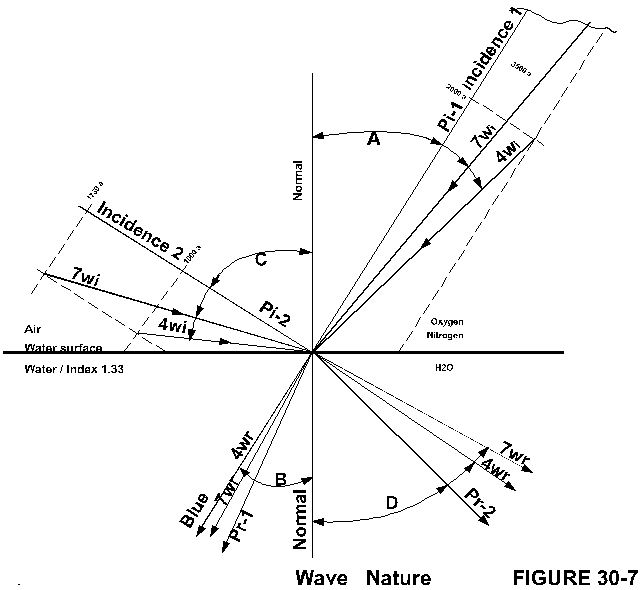1. Figure 30-5 showed light to pass into water from a direction called “the normal".  By figure 30-7, let us review the incidence of light out of the normal to behold how and by what, and why they are refracted as they are.

2. The rule is that the incidence entering the denser media will bend to the normal, and that the index of refraction is found by dividing the sine of the angle of incidence by the sine of the angle of refraction.  And the angle of refraction is found by dividing the sine of the angle of incidence by the index of refraction.

3. The common theory being that since blue waves travel slower in water than the red waves, that therefore they take a greater turn by which the waves become separated to behold the individual colors.

4. Illogical as this deduction may be in the cause to the separation of the individual waves.  There is also this problem with the computation in the angle of refraction by which the light is given to strike out of the normal, since they do not correspond with the angle of the incidence at which the light factually strikes the surface.

5. And to illustrate the latter; incidence Pi-1 figure 30-7 is indeed the angle of incidence at which the light comes to the surface, and by the before mentioned formula comes to a refractive angle noted Pr-1.

6. But the individual wavelengths of light do not correspond to that angle, since that line, in that angle, is merely the primary incidence at which all of the wavelengths in parallel fashion approach the surface.

7. Each individual wave within that angle of incidence has its own angle of incidence according to the length thereof, which for the red at 7000a is noted 7wi, and for the blue 4wi.  Each of which respectively refracts to 7wr, and 4wr, the red in this case by the computation taking the shorter turn instead of the blue towards the normal (tangent B).

8. The difference in pi and wi may be fractional and is too minute to be determined by eyesight, yet it nonetheless exists.

9. For our benefit the illustration being near to scale - shows only the diameter greatly exaggerated.  Incidence 2, noted Pi2 again is no more than the primary incidence (Pi) within which the red and blue are shown to their refractive angles 7wr and 4wr, with blue taking the shorter turn towards the normal.

10. Does it now seem that the shorter waves take a more acute angle of refraction than their greater cousins?  Of course not, the index of the water for refraction is the same for any wave, and not selective to size.

11. The only reason the blue takes a diverging direction of propagation at a shorter turn than the red is because it strikes the surface at a greater angle to the red.  (Tangent C, to tangent D)

12. When a ray of light comes by any incidence its wavelengths travel a parallel course (pi-2), presenting the line we now call;  “the primary incidence” (pi), within which each wave presents its own incidence in the length thereof.

13. The primary incidence is thus the same as arrows Z in figure 30-3, and the movement in relative velocity AB, or CD in figure 30-5.  While the “wave-form incidence” (wi) of figure 30-7 is the same as arrow x or y in figure 30-3, and as AG, or GB, or CF in figure 30-5, - always remembering that waves come by a twofold factor.

14. Diverging wavelengths thus are by equal or proportional angles of refraction, each according to their own incidence.  If this were not the case our spectra would not show its band of colors, nor would the shift to the one side or the other be recorded upon it.

15. Accordingly, we conclude with two angles of incidence, primary and waveform, or single incidence in twofold.  And that the dispersion of the diverging beams is proportional to the angular difference in the incidence, with the cause being “angle of incidence” rather than velocity.

Wave shift1. I began stating that we were going to learn more about waves, but come to know less than before.  For the more we learn the more seems to remain unanswered.

2. One of these has to do with the spectra, the shift in wavelength verses speed.  Record shows the nearest galaxies to have the smallest red shift and more distant galaxies the largest red shift, suggesting the more distant the faster these galaxies move away from us.

3. I of course am not one to believe in a contracting or expanding universe, or the big bang or what all, since I know better.  Yet there has to be an answer for this phenomena, that I have yet to put my finger on.

4. I am sure it is something very simple, but however simple a mystery remains a mystery.  And while I do not have experimental tools at my disposal, nor being in a position to physically scrutinize the tools others have unto themselves that answer for the time being remains pending.

5. The record shows Virgo at a radial velocity of 1200km/sec to Hydra as much as 61,000km/sec all by a red shift H + K.  {I hope the astronomers had enough sense to consider our own velocity as part of any red shift)

6. At the same time the record also shows that in order for light to have a relative velocity of 299.910 km/sec in the longest to 299,725 km/sec for the shortest lengths, that the angular component from one to the other cannot be more than from 2.096a to 3.667a (Figure 30-8).

7. Simply meaning that if we expand a red wavelength by 2.096a it becomes a straight line.  Or meaning, we cannot impose a greater red shift upon a 7000a wavelength than the sum total of its angular component, which for the circular wave train at a diameter of 54.56a is 54.56a in 2.096a, equal to 90km/sec.

8. By illustration figure 30-8 where AB is the linear component, with ACB the linear and angular combined.  A partial red shift noted A/C1/B1 to place one half of the 2.096a angular component into the linear would give us a red shift of no more than 45km/sec, or, for the 3.667a of the blue wave a velocity no more than 137.5 km/sec.

9. And for the maximum, to pull the wave into a straight line (Figure 30-8-B2), the 4000a wave would give us no more than a 275-km/sec reading.

10. These figures then being factual in the measurement of the velocity of light, and of its wavelengths, and of the constant by the assumption of being not more than 300,000 km/sec, how by a red shift reading did we come to the radial velocities as mentioned of the galaxies?

11. There is plenty of lead-way in shifting to the blue, namely, the whole length of the wave to fold it into a vertical line if necessary whereby the velocity of the linear component comes to zero.  But in a shift to the red, what is straighter than a straight line?

12. Then there is the fact that all wavelengths are to be seen by their half measure, This is so by virtue of their angular moment around a circumference.

13. One alternate might be to suggest that after a 90km/sec receding velocity the wave having become no more than a line - that like unto a rubber band it is stretched.

14. All good and well, but if a line cannot be read for its velocity how do we read a stretched line for its velocity?  And if it were stretched - what is that to its signature, its identity?

15. By figure 30-8 - if C were the vector and point B upon our spectrum the normal, with points x, y, z, the shift into the red, there is no greater shift than point z.

16. There is of course this alternate, that we are in error regarding our relative velocity of light, or the amplitude, the diameter, which if it were larger, the angular component would be larger.  Or that we are in error regarding the constant, that it is not 300,000km/sec.

17. For again, this thing is a fact, that in the example of the 7000a wave I am not in error with either the identity at 7002.096, nor with the diameter at 54.56a.  Since these are simply the mathematical solutions taken from the experimental data of wavelength 7000a, Vr 299,910 km/sec, and Vc 300.000 km/sec.

18. And so while I do not doubt that our spectra are actual readings for these galaxies to wit, Virgo, Ursa Major, Corona Borealis, Bootes, and Hydra, I am as yet in the dark to explain how they got there, or how to justify the consequential velocities.

19. In my judgment I sense an error somewhere in the shift, as also in the greater to greater radial velocity.  It simply does not seem to make sense, but where to find the error, or alternate.

20. Before all this however it seems prudent to first find some answer for the red shift, which by our current predicament cannot be larger than at best a few hundred kilometers per second.  Perhaps if we proceed with more of in the nature of light something will catch our eye.

Refraction how?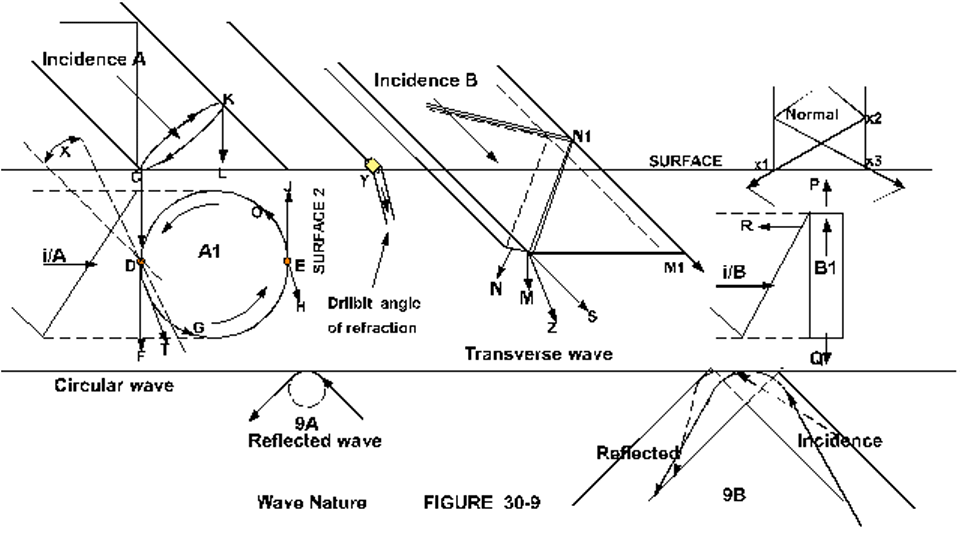1. Now that we know the cause to the dispersion in refracted light, the question becomes how and why refraction occurs.  The typical example of wave fronts perpendicular to the line of incidence no doubt is plausible, except that our waves are more like vertical rods, or drill-bits instead of perpendicular fronts.

2. If we consider a transverse wave incidence B by figure 30-9, the natural direction for a projectile, or a straight line would be direction S.  But the object being a wave strikes the surface out of center or out of its equilibrium.

3. That first contact point then tends to dig in to three simultaneous directions S, M, and N resulting into a net outcome roughly into the direction Z.   But the wave being transverse should it not dig more into direction N than what the composition in the index thereof calls for - by the frontal version of refraction?

4. If this were the case, viewing the same wave from the top by B1 at a quarter turn to B, there would be an angular movement towards either P or Q in addition to the normal refracted angle Z.

5. Or supposing part N1 of incidence B contacts the surface first at point M1, would then the wave by the frontal version refract into a direction opposite to N?

6. For in the case of the wave coming from the normal, and X1 came first, it would dig in as indicated, and x2 coming at x3 the right side would dig in as the left had done - the wave proceeding in its linear normal direction with a greater amplitude.  Here again, I think we need to experiment.

7. I judge the cause in the refraction of magnetic waves to simply be a mechanical one.  In the case of the circular wave incidence figure 30-9-A, point C as the first point on the circumference striking the surface - digs into the composition to which there are two simultaneous factors.

8. The wave being resisted in its downward movement - not instantaneous at all points, tends to receive a force to drive it out of its incidence applying more force towards K to move towards L.

9. Professional experience having shown that when drilling into wood with a long flexible drill-bit (Figure 30-9-Y), that if the operator does not counteract the drill - the bit will refract into the wood like unto a wave into a denser media.

10. This however for the wave of light does not occur, for several reasons.  Number one is it flexibility to change, and number two the factor of its inertia, the inertia maintaining the incidence.

11. Following through on the circular wave, the force striking at point D, (top view of C) will tend to head in direction F that is into the paper rather than the arrow.  And the wave moving counter-clockwise will proceed into G to the net outcome in the general direction of T.

12. And since the wave must expand for the greater density of the composition - the circular movement into G will proceed by a greater diameter, which in turn reduces the relative velocity of the wave.

13. The chances of a wave striking first at point C, or D, is of course only 1 in 366 for a circle of 366 degrees.  And so what if it strikes first at point E?  The scenario at E would be the same for point D; it digs into the paper by J, with O the direction of rotation, the net outcome of which is near H, the same direction as T.

14. And the same applies for any point on the circumference, since the wave is a circular wave for which reason it will always refract into the same angle regardless to what part of the wavelet strikes first.

15. Here the circular wave version varies from the transverse version if indeed a transverse wave refracts into two directions for its transverse nature.

16. And in the case of a reflected wave figure 30-9-A, the angle of reflection being equal to the angle of incidence, is the same for a circular as well as for a transverse wave.  Or even for no wave at all - as with sound where a vibration will reflect equal to its angle of incidence.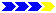To the second half of "Wave spectrum"